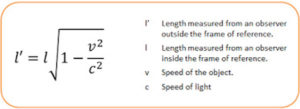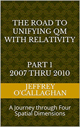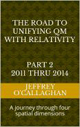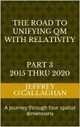Entanglement provides a VERY SIMPLE experimental way of determining if Quantum mechanics or Einstein’s Relativistic theories define why our universe is what it is.

This is because it is one of the central principles of quantum physics. In short it assumes two particles or molecules share on a quantum level one or more properties such as spin, polarization, or momentum. This connection persists even if you move one of the entangled objects far away from the other. Therefore, when an observer interacts with one the other is instantly affected.However, it contradicts the central core Einstein’s theory of Relativity which states that no information can be transmitted instantaneously or faster than the speed of light.

Since these two concepts are diametrically opposite, if one can define the mechanism responsible for entanglement in terms of either one it would invalidate the other will help us to understand why our universe is what it is.

This is because there is irrefutable experimental evidence the act of measuring the state of one of a pair of photons instantaneously affect the other even though they are physically separated from each other.

As was mentioned earlier quantum physics, assumes ALL entangled particles, not only photons remain connected so that actions performed on one immediately affect the other, even when separated by great distances.

While Einstein tells us that instantaneous or faster than light communication between to particles is impossible.  However, he also told us the distance between two objects or points in space is defined by their relative motion and that there is no preferred reference frame by which one can define that distance.

Therefore, he tells the distance between the observational points in a laboratory, can also be defined from the perspective of the photons moving at the speed of light.

Yet, his formula for length contraction (shown below) tells us the separation between those observational points from the perspective of two photons moving at the speed of light would be ZERO no matter how far apart they might be from the perspective of an observer in that laboratory. This is because, as was just mentioned according to the concepts of Relativity one can view the photons as being stationary and the observers as moving at the velocity of light.

Therefore, according to Einstein’s theory all photons which are traveling at the speed of light are entangled no matter how far they may appear to be someone who is looking at them.  Additionally, it also tells us information exchange between two entangle photons does not travel faster than the speed of light because from their perspective the distance between the observation points where information was read is zero.

In other words, entanglement of photons can be explained and predicted terms of the relativistic properties of space-time as defined by Einstein as well as by quantum mechanics.

HOWEVER, AS WAS MENTIONED EARLIER ONE OF THE CORE PRINCIPALS OF QUANTUM MECHANICS  IS THAT ALL PARTICLES SHARE ON A QUANTUM LEVEL ONE OR MORE PROPERTIES SUCH AS SPIN POLARIZATION OR MOMENTUM.

This gives us a way of experimentally determining which of these two theories define why entanglement occurs because if it is found that some particles that are NOT moving at the speed of light experience entanglement it would validate one of the core principals of quantum mechanics and invalidate Relativities assumption that information cannot be exchange instantaneously or faster that the speed of light.

However, one MUST ALSO use another core principle of quantum mechanics defined by De Broglie that particle are made up wave with a wavelength defined by ? = h/p to determine if it or Einstein’s theories define how the universe works. This is because it tells us all material particles have an extended volume equal to there wavelength

Yet because ALL particles have an extended volume equal to their wavelength there will be an overlap or entanglement if the distance separating them is less than their volume as defined by De Broglie.

This tells us some particles moving slower than the speed of light CAN BE entangled if the relativistic distance between the observation points from the perspective of the particles is less than their extended volume is because from their perspective they are in physical contact.

This means that both relativity and quantum mechanics tell us that all particles CAN be entangled if the distance between the end points of the measurements of their shared properties is less than their wavelength or volume as defined by De Broglie.

However, this gives us a way to DEFINITIVELY determine which one of these theories defines the reason for entanglement because we can precisely define the wavelength and therefore the volume of a particle by, as mentioned earlier using De Broglie formula ? = h/p while one can determine the relative distance between the observation points from the perspective of the particles being observed by using Einstein formula for length contraction.  If it is found entanglement DOES NOT occur if that distance is greater than a particles volume then it would invalidate the core principles of quantum mechanics that two particles or molecules share on a quantum level one or more properties such as spin, polarization, or momentum no matter how far they are separated.  However, if it is found that entanglement does occur even if the separation was greater than their volume it would invalidate the core principals of relativity that no information can be transferred faster that the speed of light.

In other words, it gives us a doable experimental that will UNEQUIVOCALLY tell us if Quantum Mechanics or Einstein’s’ theories define why the universe is what it is

IT CANNOT GET MUCH SIMPLER THAN THAT.

 The Road to Unifying QM with Relativity part 1 2007 thru 2010Ebook \$8.00 The Road to Unifying QM with Relativity part 2 2011 thru 2014Ebook \$8.00 The Road to Unifying QM with Relativity part 3 2015 thru 2020Ebook \$8.00